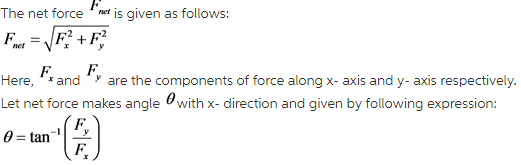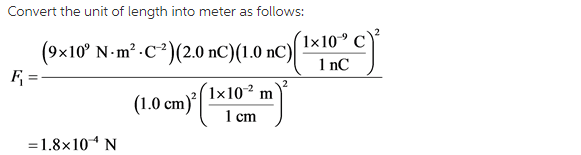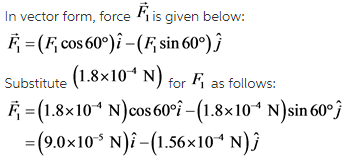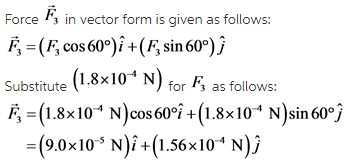# What is the force F? on the 1.0 nC charge in (Figure 1) ? Determine the magnitude of the force F?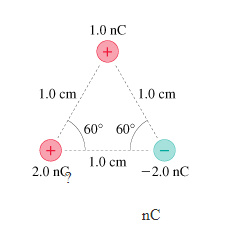What is the force F? on the 1.0 nC charge in (Figure 1) ?

Determine the magnitude of the force F?
Determine the direction of the force

Concepts and reason
Electrostatic force, sometimes known as Colombian force is the attraction and repulsion between two objects carrying net charge. It can be attractive or repulsive in nature depending upon the charge involved.
For like charges, the force is repulsive and between unlike charges the force is attractive in nature.

Fundamentals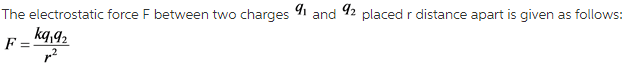Here, k is the proportionality factor and possess a constant value for a medium.